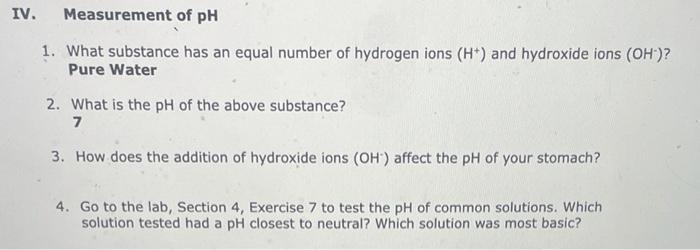Home / Expert Answers / Biology / iv-measurement-of-ph-1-what-substance-has-an-equal-number-of-hydrogen-ions-left-mathrm-h-pa946

# (Solved): IV. Measurement of pH 1. What substance has an equal number of hydrogen ions $$\left(\mathrm{H}^{+ ...IV. Measurement of pH 1. What substance has an equal number of hydrogen ions \( \left(\mathrm{H}^{+}\right)$$and hydroxide ions $$\left(\mathrm{OH}^{-}\right)$$? Pure Water 2. What is the $$\mathrm{pH}$$ of the above substance? 3. How does the addition of hydroxide ions $$\left(\mathrm{OH}^{-}\right)$$affect the $$\mathrm{pH}$$ of your stomach? 4. Go to the lab, Section 4, Exercise 7 to test the $$\mathrm{pH}$$ of common solutions. Which solution tested had a pH closest to neutral? Which solution was most basic?

We have an Answer from Expert